# Complete Riemannian space

(diff) ← Older revision | Latest revision (diff) | Newer revision → (diff)
A Riemannian space with its internal distance functionthat is complete as a metric space with metric.
Letbe a connected Riemannian space with its Levi-Civita connection, then the following three assertions are equivalent: a)is complete; b) for each point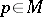the exponential mapping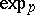is defined on all of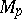(whereis the tangent space toat); and c) every closed setthat is bounded with respect to the distanceis compact (the Hopf–Rinow theorem). Consequences are: Any two points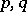in a complete Riemannian spacecan be joined inby a geodesic of length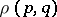; any geodesic is indefinitely extendable.